# Converting Data Types with VBA Functions

 < Day Day Up >

At some point, you'll probably have to import data from a foreign source or you might be forced to use existing data in a manner not originally intended. When this happens, you might need to convert data from one data type to another, and VBA provides many functions for converting data types. Just remember that you're not changing the stored value's data type.For a more in-depth review of the VBA data types, read "VBA Data Types," in Chapter 3 (p. 39).

For a complete list of functions that force a specific data type, open the Help task pane in the VBE and search for "Type Conversion Functions." In this chapter, you learn how to use several of the most common conversion functions:

• CBool Converts a value to a Boolean data type.

• CByte Converts a value to a Byte data type.

• CDate Converts a value to a Date data type.

• CInt Converts a value to an Integer data type.

• CStr Converts a value to a String data type.

• CVar Converts a value to a Variant data type.

TIP

There are a few older conversion statements, such as Str, still included in the most recent versions of VBA. The most up-to-date conversion functions start with the letter C. It's best to use these later functions because they consider your system's date, time, and number settings, whereas the older statements don't.

The conversion functions all use the same simple syntax

` `

` CBool(variable) `

where variable is the name of a variable, constant, or an expression that evaluates to a specific data type. The functions convert variable to the appropriate data type so you can use the converted value elsewhere. Nothing happens to the stored value. However, not every data type can be converted to any other data type. You learn more about these conversion pitfalls in the following sections dedicated to specific functions.

#### Converting to a Boolean Data Type

Use CBool to convert a value to a Boolean data type. The variable argument is required and can be a string or a number. You might think that means the value being converted must be implicitly or explicitly, 1 or 0, but the value being converted can be any number or string that can be interpreted as a number.

When the value is a numeric or string 0, CBool returns False. Any other value or string returns True ( 1). For instance, all the following expressions return True because variable can be interpreted as a numeric value and that value is a number other than 0.

` `

` CBool("1") CBool(1+0) CBool(2) CBool(-300) `

On the other hand, both of the following expressions return False. In both cases, variable is interpreted as the numeric value 0. Therefore, both return False.

` `

` CBool(0) CBool("0") `

CBool can't handle every character. VBA must be able to express the value you're converting as a numeric value. That includes digits stored as strings, but not alphabetic characters. Figure 5.1 shows such an example in the Immediate window returning a mismatch data type error. The expression CBool("one") returns a mismatch error because VBA can't interpret the string "one" as the numeric value 1.

##### Figure 5.1. CBool returns an error when VBA can't interpret a numeric value.CAUTION

CBool converts any value or expression that evaluates to 0 to False and any non-zero value to True. Consequently, a False value can be converted back to its original value of 0. A True value can be restored to its original value only when it was originally 1.

#### Converting to a Byte Data Type

The Byte data type stores numeric values from 0 to 255. The variable argument is required and can be a Numeric or String data type. CByte converts a value from 0 to 255 or any variable or constant that can evaluate to a value from 0 to 255 to the Byte data type. The following functions return the Byte values 0, 255, and 1, respectively:

` `

` CByte(0) CByte(255) CByte("1") `

When the value being converted is out of the Byte data type's range (from 0 to 255), CByte returns an overflow error message, as shown in Figure 5.2.

##### Figure 5.2. A value out of the Byte range generates an overflow error.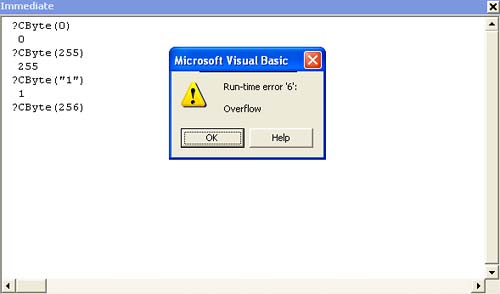TIP

CByte rounds a floating point number to the nearest integer before converting to the Byte data type. As long as the rounded integer is from 0 to 255, CByte converts it.

#### Converting to a Date Data Type

The CDate function converts a value to a Date data type. The variable argument is required and the value can be a String or Numeric data type or any valid date expression. CDate uses your system's locale settings to determine the order of the three date components, day, month, and year. The following guidelines might help when converting to the Date data type:

• CDate converts the integer portion to the date represented by the same number of days that have passed since December 31, 1899. The fraction is converted to the time of day, where .01 represents 14 minutes and 24 seconds (864 seconds) past midnight.

• CDate accepts both numeric and string values. For instance, CDate can correctly interpret the following string values: "3/1/04", "March 1, 2004", "1 Mar 04", and 38046 (the date serial value equal to March 1, 2004).

• The Date data type supports dates from January 1, 100 to December 31, 9999. Any value out of that range generates an error.

• Two-digit year values that are less than 30 are converted to 21st century dates. Year values that are greater than or equal to 30 are converted to 20th century dates. When the year value is omitted, CDate uses the current year.

Figure 5.3 shows the evaluated result of a CDate function that returns an unexpected result. CDate interprets 3/1/04 as an equation and works the math as follows (because there are no parentheses, the equation is evaluated from left to right):

` `

` 3/1 = 3 3/04 = .75 `

##### Figure 5.3. Be sure to properly delimit a date string.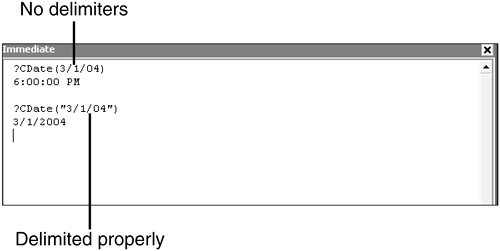The resulting fractional value of .75 is processed as a time value where .75 is three quarters through the day, or 6:00:00 PM. The key here is to remember to delimit a date string as a string. Then, CDate will convert the date value properly.

#### Converting to an Integer Data Type

Use CInt to convert a numeric or string value to an Integer data type. The variable argument is required and its range can be any variable, constant, expression, or literal value from 32,678 to 32,767. When the fractional component is exactly 0.5, CInt rounds to the nearest even number. For example, 0.5 rounds to 0 and 1.5 rounds to 2. Both of the following functions return the integer value 1000:

` `

` CInt(1000) CInt("1000") `

If variable evaluates to anything other than a numeric value, the function returns an error. If variable evaluates to a numeric value outside of the Integer data type range, the function generates an overflow error.

TIP

CInt is more flexible than the Val function because it uses the system's regional settings and can recognize the thousands separator. For instance, CInt correctly converts a String value of "1,234" to 1234. Val converts the same value to 1.

#### Converting to a String Data Type

Use CStr to convert almost any value to a String data type. The variable argument is required, but it can equal any variable, constant, expression, or literal value that can evaluate to a string. Although that covers a lot, prepared for a few unexpected results:

• Uninitialized Numeric data types return "0"

• Unintialized Date data types return "12:00:00 AM"

TIP

The term uninitialized describes a variable that has not been defined by a value. In other words, it's a variable's state between the time it's declared and when you give it a value to store.

#### Converting to a Variant Data Type

The section "VBA Data Types" in Chapter 3 introduces the Variant data type as the most versatile because it can store almost any value. Using CVar, you can convert almost any numeric or string value to the Variant data type. Numeric values are restricted to the same range as the Double data type; there are no restrictions on non-numeric values.

CAUTION

It's best to use CVar only when the data type isn't important (which is almost never) or when there's no doubt as to the value's data type.

#### Converting Null Values

Few functions can handle a Null value, so if a variable is Null, it's probably going to cause you trouble even though a Null value isn't inherently incorrect. For instance, the simple equation

` `

` varResults = value1 + value2 `

returns a runtime error if either value1 or value2 is Null.

You can avoid runtime errors caused by Null values by using the Nz function to convert the Null value to 0, a zero-length string (""), or a specific string value. After wrapping both value1 and value2 in an Nz function as follows, the expression no longer returns an error when value1 or value2 is Null:

` `

` varResults = Nz(value1) + Nz(value2) `

The Nz function uses the form

` `

` Nz(value, [valueifnull]) `

where value is any data type that represents the evaluated value or expression and valueifnull is the value you want the Nz to return when value is Null. If the optional argument is omitted, Nz returns either 0 or a zero-length string, based on value's data type.

##### A Conversion Example

Now let's look at a quick example that solicits a date from a user and then performs some simple date arithmetic. Suppose users need a rough estimate of the number of days to complete a new project that's starting today. To get started, launch the VBE by pressing Alt+F11. Then, complete the following steps:

1. Open a new blank module by choosing Insert, Module.

2. Next, enter the following procedure:

` `

` Public Sub GetDate()   Dim varDate As Variant   varDate = InputBox("Please enter date")   MsgBox Now - varDate End Sub `Learn how to enter a function procedure in "Entering and Running VBA Code," in Chapter 2 (p. 22).

1. With the insertion point inside the procedure, press F5 to run the code.

2. When the input box shown in Figure 5.4 appears, enter a date using any valid date string and click OK.

##### Figure 5.4. Enter a valid date string.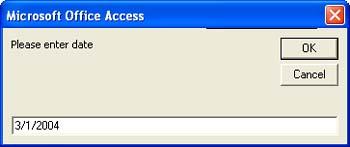3. When VBA displays the mismatch error, click Debug to return to the procedure. Figure 5.5 shows the line where the error occurs VBA highlights it.

##### Figure 5.5. The MsgBox line is returning an error.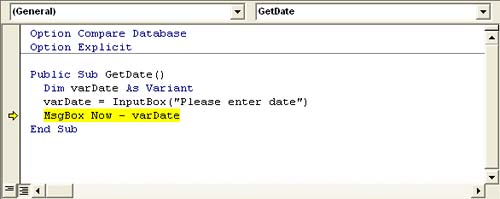4. Click Reset on the standard toolbar.

NOTE

A few of the examples use the VBA InputBox function to display a dialog box that prompts the user to enter data. Like the MsgBox function, you'll see this function several times in this book. In its simplest form, this function requires only the prompt text that the dialog box displays to remind the user what type of data is needed. The function returns that variable as a Variant String subtype. In this context, a subtype is simply one of several data types that Variant accepts.

The problem is the equation that subtracts your date string from the Now function, which returns the current date. VBA can't do the math because the variable that's storing the input date is a Variant. VBA needs a Date data type to do the date arithmetic. One way to eliminate the error is to convert the passed data.

1. Between the InputBox line and the MsgBox line, enter the function

` `

` varDate = CDate(varDate) `

2. With the insertion point in the procedure, press F5.

3. Enter the same date in the input box and click OK.

4. This time the MsgBox can return the results of the simple equation Now varDate, as shown in Figure 5.6.

##### Figure 5.6. After changing the data type of the input date string value, the equation works.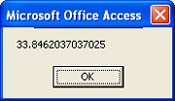5. Click OK to clear the message box and save the module, if you like, as Chapter5.

After you change the date string's data type from Variant to Date, VBA can calculate the number of days between the two dates. There's a simpler way to solve the problem.

Instead of declaring varDate as a Variant, declare it as a Date to begin with. Then you won't need to convert the input date value because VBA forces the data type for you.

Solutions won't always be that simple because changing a variable's data type is seldom convenient or practical. In fact, most of the time, you will not want to do so, unless you can determine, as in this case, that the variable is simply not the most appropriate data type to begin with.

 < Day Day Up >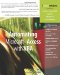Automating Microsoft Access with VBA
ISBN: 0789732440
EAN: 2147483647
Year: 2003
Pages: 186